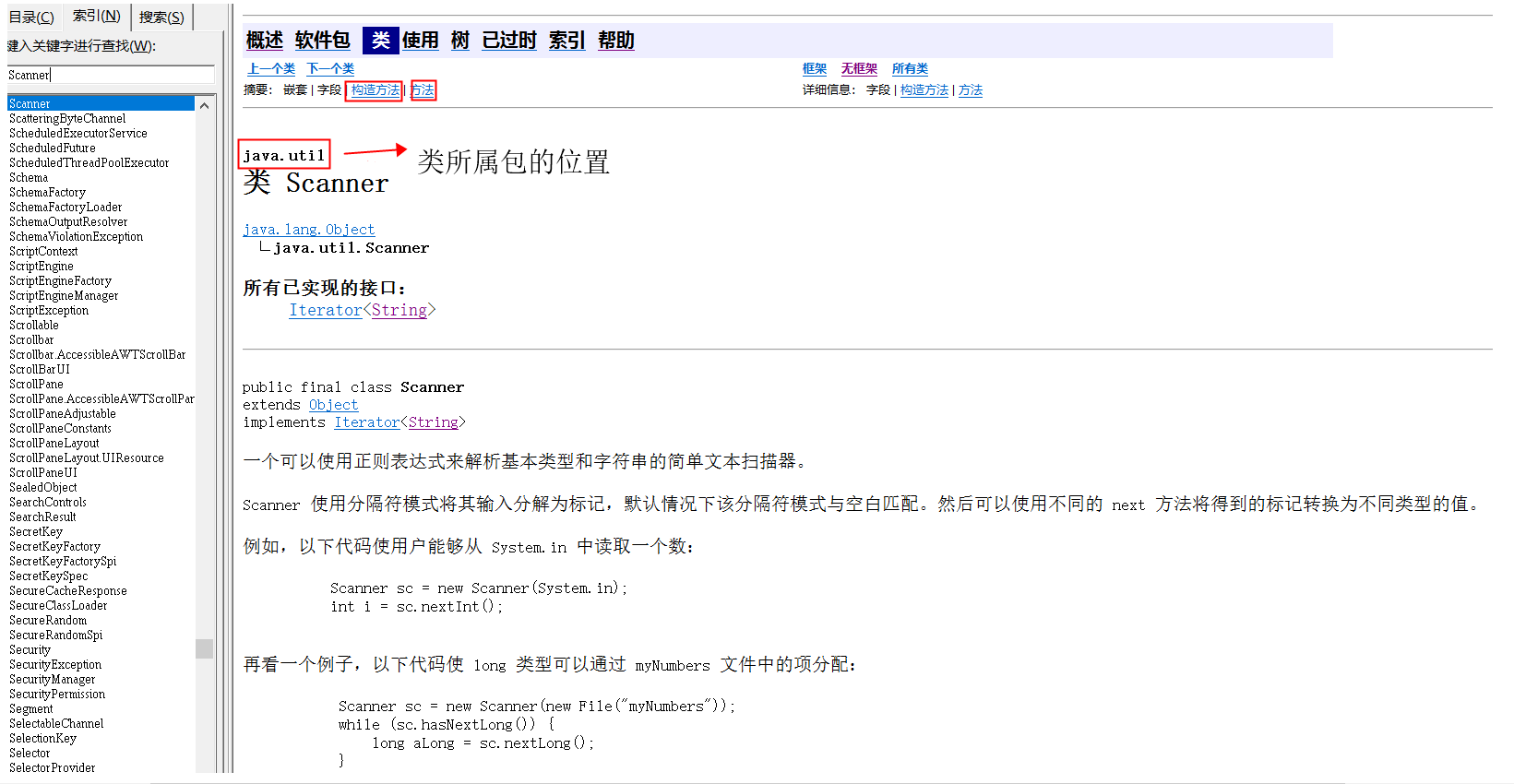## 引言

#### API的概述

API，应用程序编程接口。java API是一本程序员的字典，是JDK中提供给我们使用的类的说明文档。这些类将底层的代码实现了封装，我们不需要关心这些类是如何实现的，只需要关心这些类是如何使用即可。(文末的上传附件好像是个白名单 API上传不了 大家可以自己去搜一下API的1.6版本 是官方发布的中文版 1.6以上版本都没有官方中文版)

#### API使用步骤#### Scanner类

1. 导包
import 包路径.类名称;
`import java.util.Scanner;`
如果需要使用的目标类，和当前类位于同一个包下，就可以省略导包语句不写
只有java.lang包下的内容不需要导包，其他的包都需要import语句
2. 创建
类名称 对象名 = new 类名称();
`Scanner sc =new Scanner(System.in);`//System.in代表从键盘输入
3. 使用
对象名.成员方法名();
`String str = sc.next();`//获取键盘输入的一个字符串
``举例``import java.util.Scanner;`` public class Demo01{`` public static void main(String[] args){`` Scanner sc = new Scannner(System.in);`` int num1=sc.nextlnt();`` int num2=sc.nextlnt();`` int result=num1+num2;`` System.out.println(result);`` }``}``

#### Random类

1. 导包
`import java.util.Random;`
2. 创建
`Random r = new Random();`
3. 使用
获取一个随机的int数字 `int num = r.nextInt();`
获取一个随机的int数字（参数代表了范围，左闭右开区间）
`int num = r.nextInt(3)；`//输出范围 0，1，2
``import java.util.Random``import java.util.Scanner``public class Demo01{`` public static void main(String[] args){`` Random r = new Random();`` int num = r.nextInt(100);`` Scanner sc = new Scanner(System.in);`` int num1 = sc.nextint();`` while(true){`` if(num=num1){`` System.out.println(“猜对了”);`` Break;`` }else if(num1>num){`` System.out.println(“太大了”)；`` }else{`` System.out.println(“太小了”);`` }`` }`` }``}``

#### 匿名对象

new 类名称();

``import java.util.Scanner;``public class Demo02Anonymous{`` public static void main(String[] args){`` //匿名对象创建使用`` int num = new Scanner(System.in).nextInt();`` System.out.println(“输入的是”+num);`` } ``}``

## 结语

0 Reply   |  Until 2020-7-29 | 629 View